# Analyze the polynomial function f(x)=x2 + x2 - 20x. Complete parts (a) through (c). (a) Find...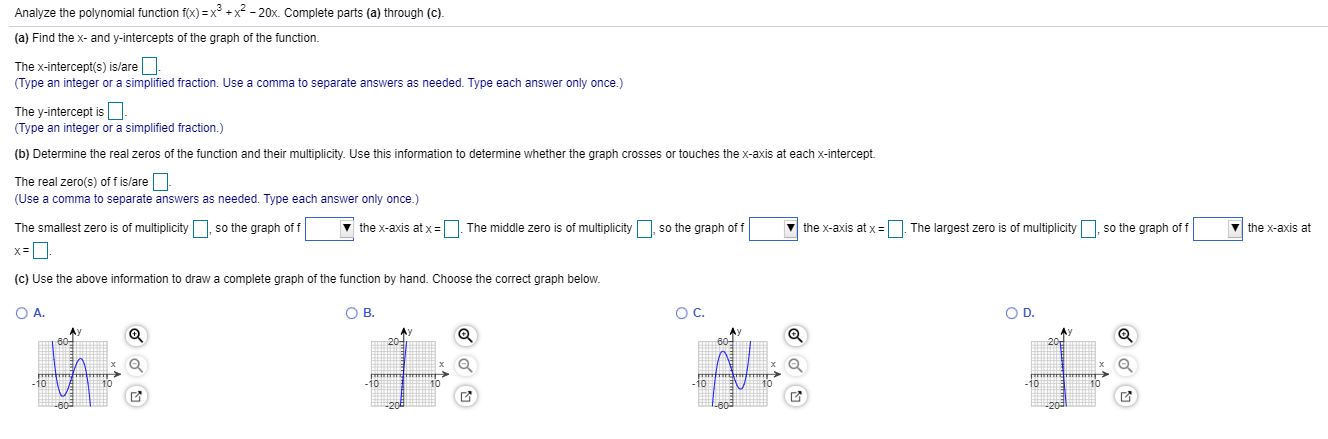Analyze the polynomial function f(x)=x2 + x2 - 20x. Complete parts (a) through (c). (a) Find the x- and y-intercepts of the graph of the function. The x-intercept(s) is/are - (Type an integer or a simplified fraction. Use a comma to separate answers as needed. Type each answer only once.) The y-intercept is - (Type an integer or a simplified fraction.) (b) Determine the real zeros of the function and their multiplicity. Use this information to determine whether the graph crosses or touches the x-axis at each x-intercept. The real zero(s) off is/are (Use a comma to separate answers as needed. Type each answer only once.) The smallest zero is of multiplicity , so the graph off the x-axis at x=. The middle zero is of multiplicity , so the graph off the x-axis at x = | The largest zero is of multiplicity , so the graph off the x-axis at (c) Use the above information to draw a complete graph of the function by hand. Choose the correct graph below. O A. OB. YO

We need at least 10 more requests to produce the answer.

0 / 10 have requested this problem solution

The more requests, the faster the answer.

All students who have requested the answer will be notified once they are available.

#### Earn Coin

Coins can be redeemed for fabulous gifts.

Similar Homework Help Questions
• ### Analyze the polynomial function tox)(x+57(4 - X) using parts (a) through a (a) Determine the end...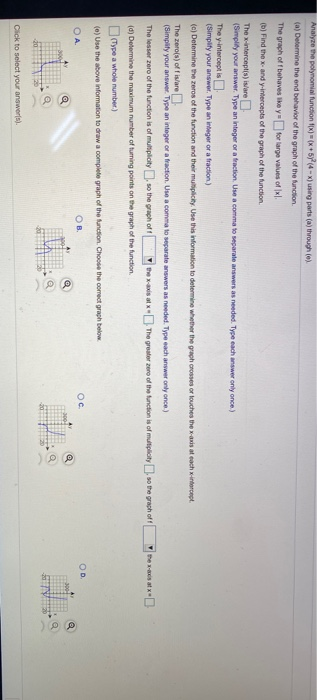Analyze the polynomial function tox)(x+57(4 - X) using parts (a) through a (a) Determine the end behavior of the graph of the function The graph off behaves ikey-for large values of lxl. (b) Find the x and y-intercepts of the graph of the function The x-intercept) is/are (Simplify your answer. Type an integer or a fraction. Use a comma to separate answers as needed. Type each answer only once) The interceptis (Simplify your answer. Type an integer or a fraction)...

• ### Analyze the polynomial function fix) = x(x 3)(x + 7) using parts (a) through (e). la...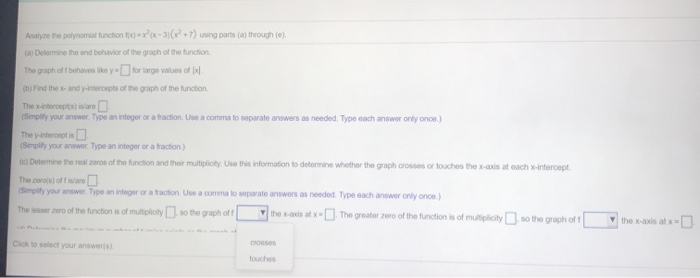Analyze the polynomial function fix) = x(x 3)(x + 7) using parts (a) through (e). la Determine the end behavior of the graph of the function The graph off behaves like y- for large values of Ix/ (b) Find the x-and intercepts of the graph of the function Thexintercepts) isare (Simply your answer. Type an integer or a tractionUse a comma to separate answers as needed. Type oath answer only once.) The interceptis (Simplify your answer. Type an integer or...

• ### Find the zeros for the given polynomial function and give the multiplicity for each zero. State...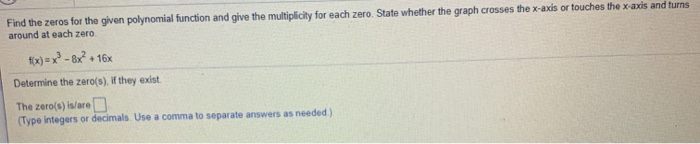Find the zeros for the given polynomial function and give the multiplicity for each zero. State whether the graph crosses the x-axis or touches the x-axis and turns around at each zero fix)=x2-8x? + + 16x Determine the zero(s). If they exist. The zero(s) is/are D (Type integers or decimals. Use a comma to separate answers as needed) Find the zeros for the given polynomial function and give the multiplicity for each zero. State whether the graph crosses the x-axis...

• ### Answer parts a.-e. for the function shown below. #x-x3-4x2-x+4 a. Use the leading coefficient tes...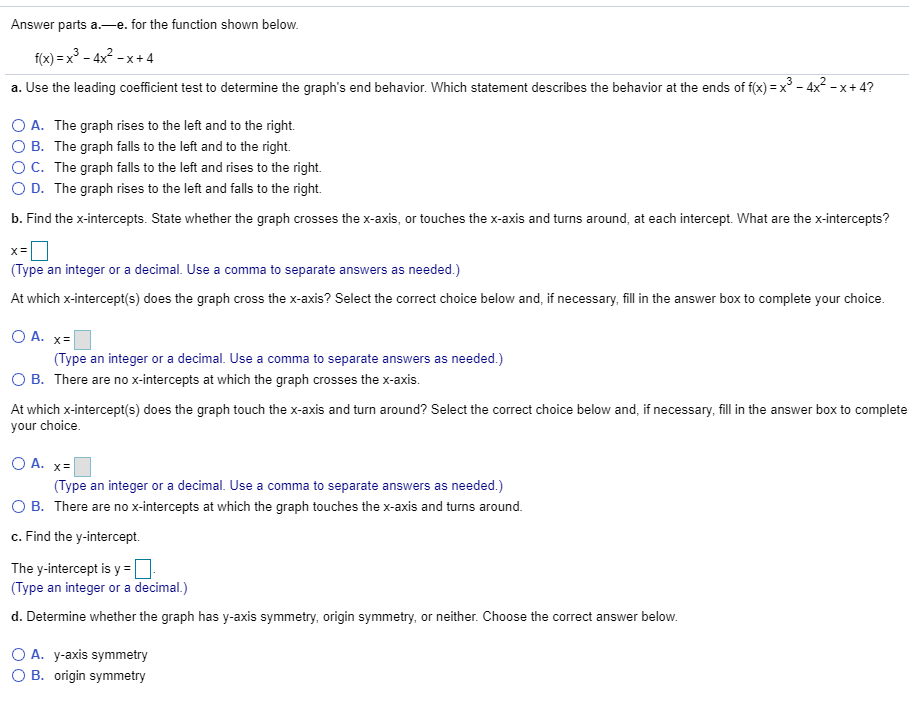Answer parts a.-e. for the function shown below. #x-x3-4x2-x+4 a. Use the leading coefficient test to determine the graph's end behavior. Which statement describes the behavior at the ends of f(x)=x"-4X-x+4? O A. The graph rises to the left and to the right. B. The graph falls to the left and to the right. ° C. The graph falls to the left and rises to the right. D. The graph rises to the left and falls to the right. b....

• ### Use the given function to complete parts a) through e) below. f(x)= - x4 + 25x2...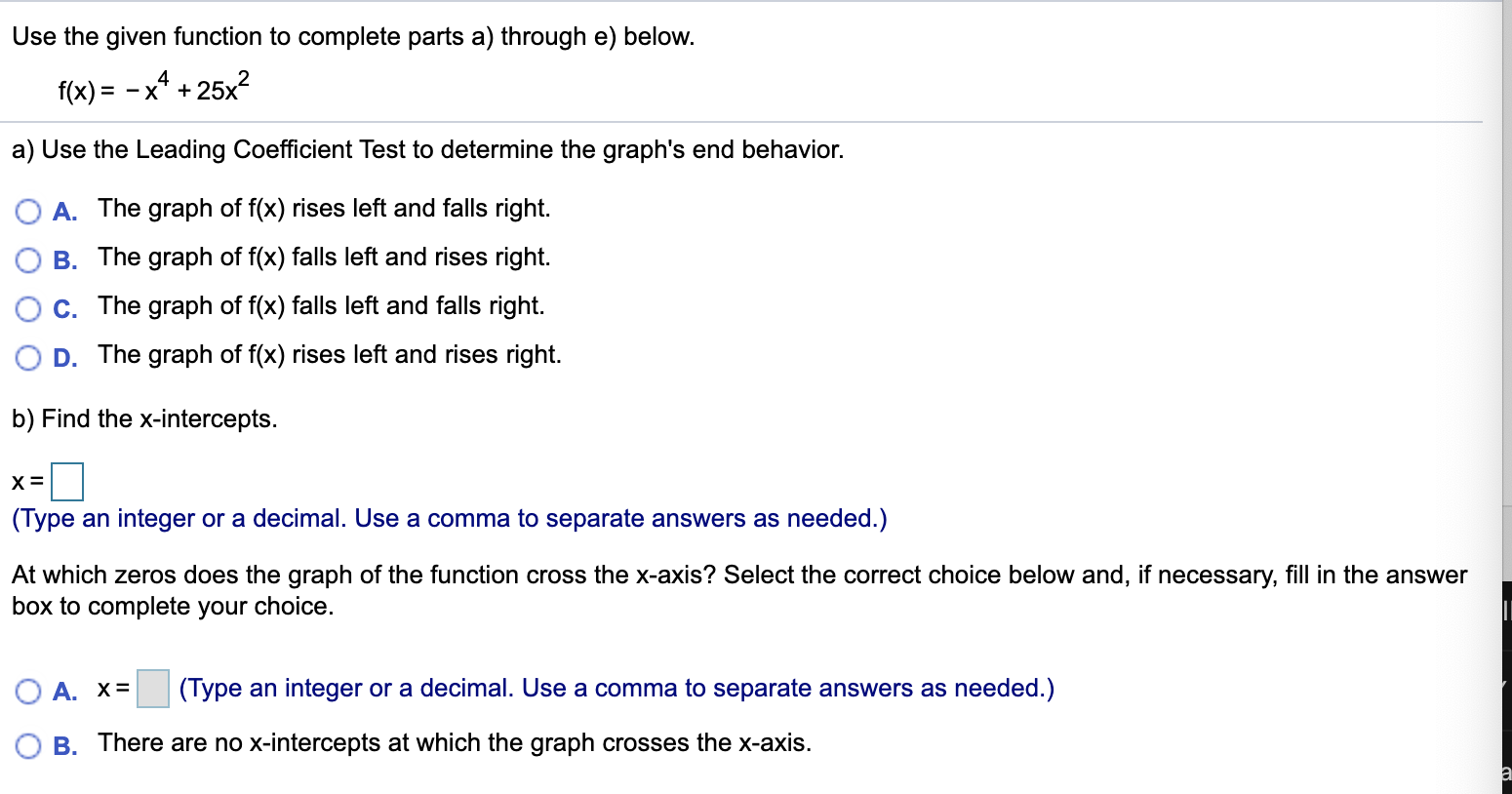Use the given function to complete parts a) through e) below. f(x)= - x4 + 25x2 a) Use the Leading Coefficient Test to determine the graph's end behavior. O A. The graph of f(x) rises left and falls right. B. The graph of f(x) falls left and rises right. OC. The graph of f(x) falls left and falls right. OD. The graph of f(x) rises left and rises right. b) Find the x-intercepts. X= (Type an integer or a decimal....

• ### Use the following function to answer parts a through a. f(x) = x2 + 4x2 -...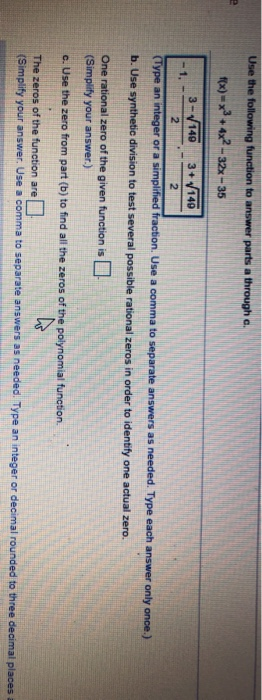Use the following function to answer parts a through a. f(x) = x2 + 4x2 - - 32x - 35 3-140 3+ 140 1. 2 2 (Type an integer or a simplified fraction. Use a comma to separate answers as needed. Type each answer only once.) b. Use synthetic division to test several possible rational zeros in order to identify one actual zero. One rational zero of the given function is (Simplify your answer.) c. Use the zero from part...

• ### 16 For the quadratic function f(x) = -x2 - 8x, answer parts (a) through (c) (a)...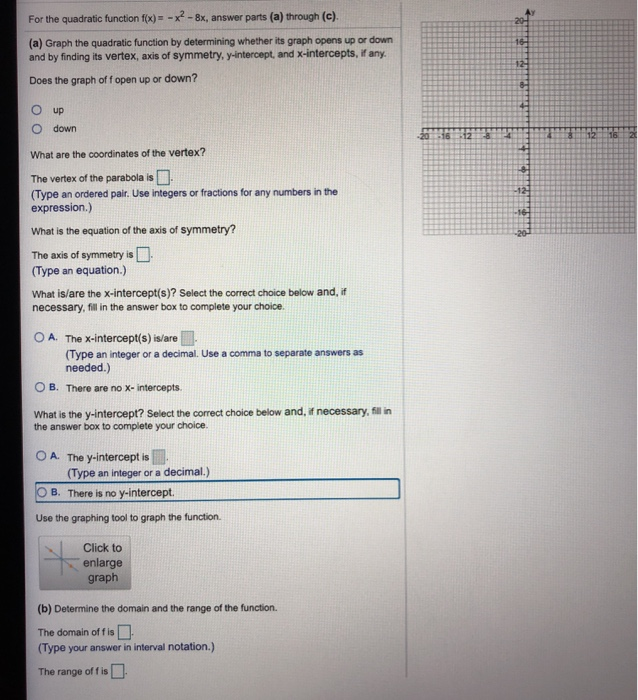16 For the quadratic function f(x) = -x2 - 8x, answer parts (a) through (c) (a) Graph the quadratic function by determining whether its graph opens up or down and by finding its vertex, axis of symmetry, y-intercept, and X-intercepts, if any. Does the graph off open up or down? 12 8- up Odown 2015 12 118 12 16 What are the coordinates of the vertex? The vertex of the parabola is (Type an ordered pair. Use integers or fractions...

• ### Follow the seven step strategy to graph the following rational function. X+2 x²+x-12 To graph the...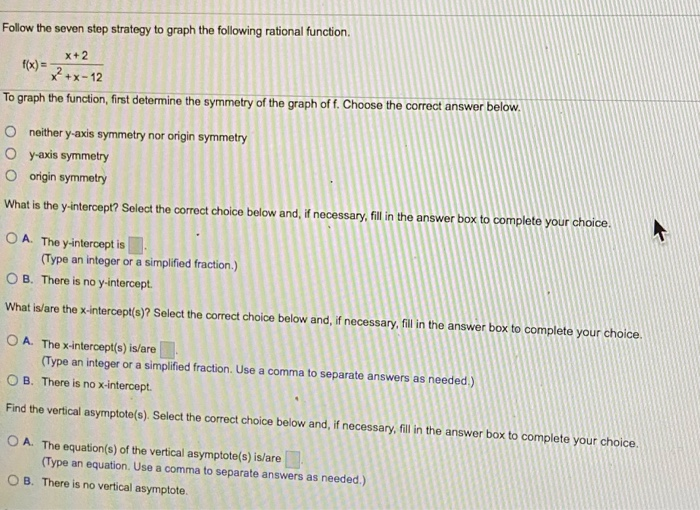Follow the seven step strategy to graph the following rational function. X+2 x²+x-12 To graph the function, first determine the symmetry of the graph off. Choose the correct answer below. neither y-axis symmetry nor origin symmetry Oy-axis symmetry O origin symmetry What is the y-intercept? Select the correct choice below and, if necessary, fill in the answer box to complete your choice. OA. The y-intercept is (Type an integer or a simplified fraction.) OB. There is no y-intercept. What is/are...

• ### can i het help with this question please The graph and equation of the function f are given. a. Use the graph to find any values at which f has a relative maximum, and use the equation to calcula...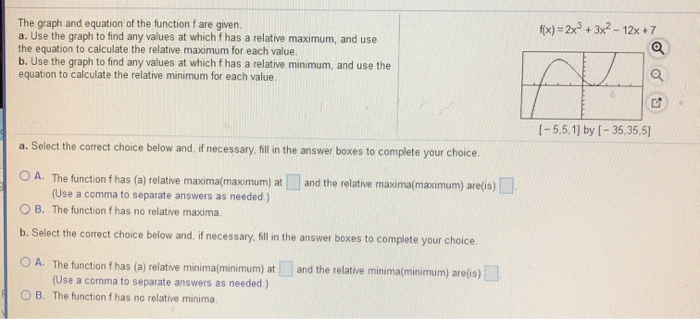can i het help with this question please The graph and equation of the function f are given. a. Use the graph to find any values at which f has a relative maximum, and use the equation to calculate the relative maximum for each value b. Use the graph to find any values at which f has a relative minimum, and use the equation to calculate the relative minimum for each value. fx)-2x3+3x2 -12x+7 I-5.5.1] by I-35,35,5] a. Select the...

• ### Use the graph shown to find the following. (a) The domain and range of the function...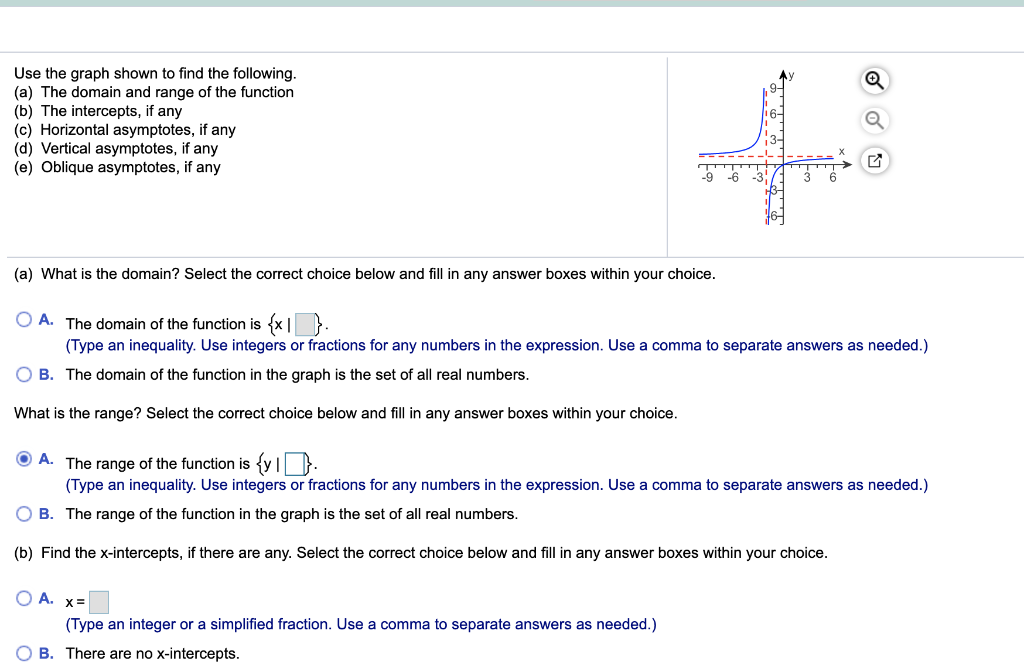Use the graph shown to find the following. (a) The domain and range of the function (b) The intercepts, if any (c) Horizontal asymptotes, if any (d) Vertical asymptotes, if any (e) Oblique asymptotes, if any 926 (a) What is the domain? Select the correct choice below and fill in any answer boxes within your choice. O A. The domain of the function is {x1 }. (Type an inequality. Use integers or fractions for any numbers in the expression. Use...